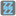## Question related to missionFizz Buzz

```def checkio(number):

if number % 3 == 0 and number % 5 == 0 return "Fizz Buzz":
if number % 3 == 0 return "Fizz":
if number % 5 == 0 return "Buzz":

return str(number)
```

the weird thing is it returns something not in my code.... don't know what happened.

Error msg:

```SyntaxError: invalid syntax (<MYCODE>, line 7)
?, ?
NameError: name 'checkio' is not defined
<module>, 1
```

Edit, 0929

I was trying to use ternary operator in python. I've found this.

http://pythoncentral.io/one-line-if-statement-in-python-ternary-conditional-operator/

<expression1> if <condition> else <expression2>

then I changed my code to:

```return "Fizz Buzz" if number % 3 == 0 and number % 5 == 0:
return "Fizz" if number % 3 == 0:
return "Buzz" if number % 5 == 0:
```

But it still not working. Anyone know what's wrong?4